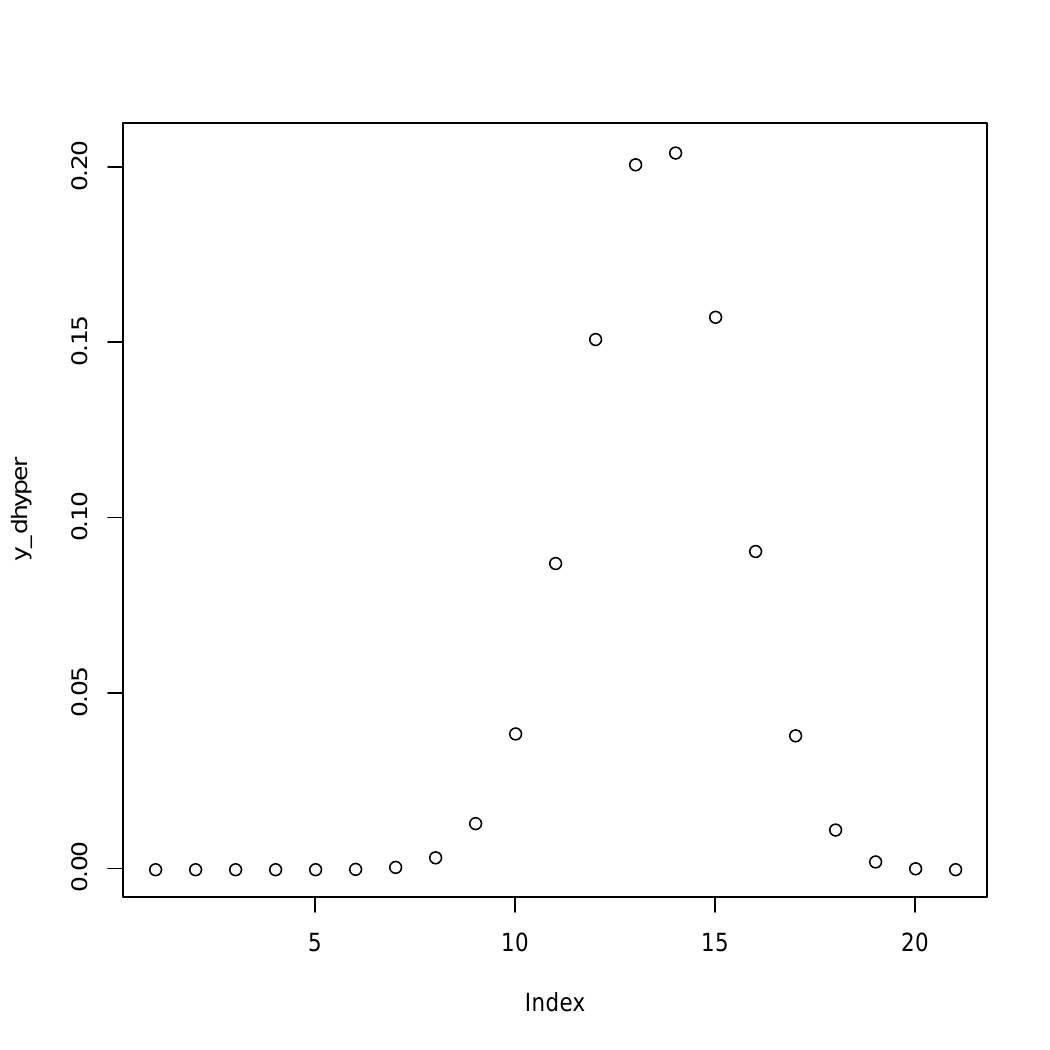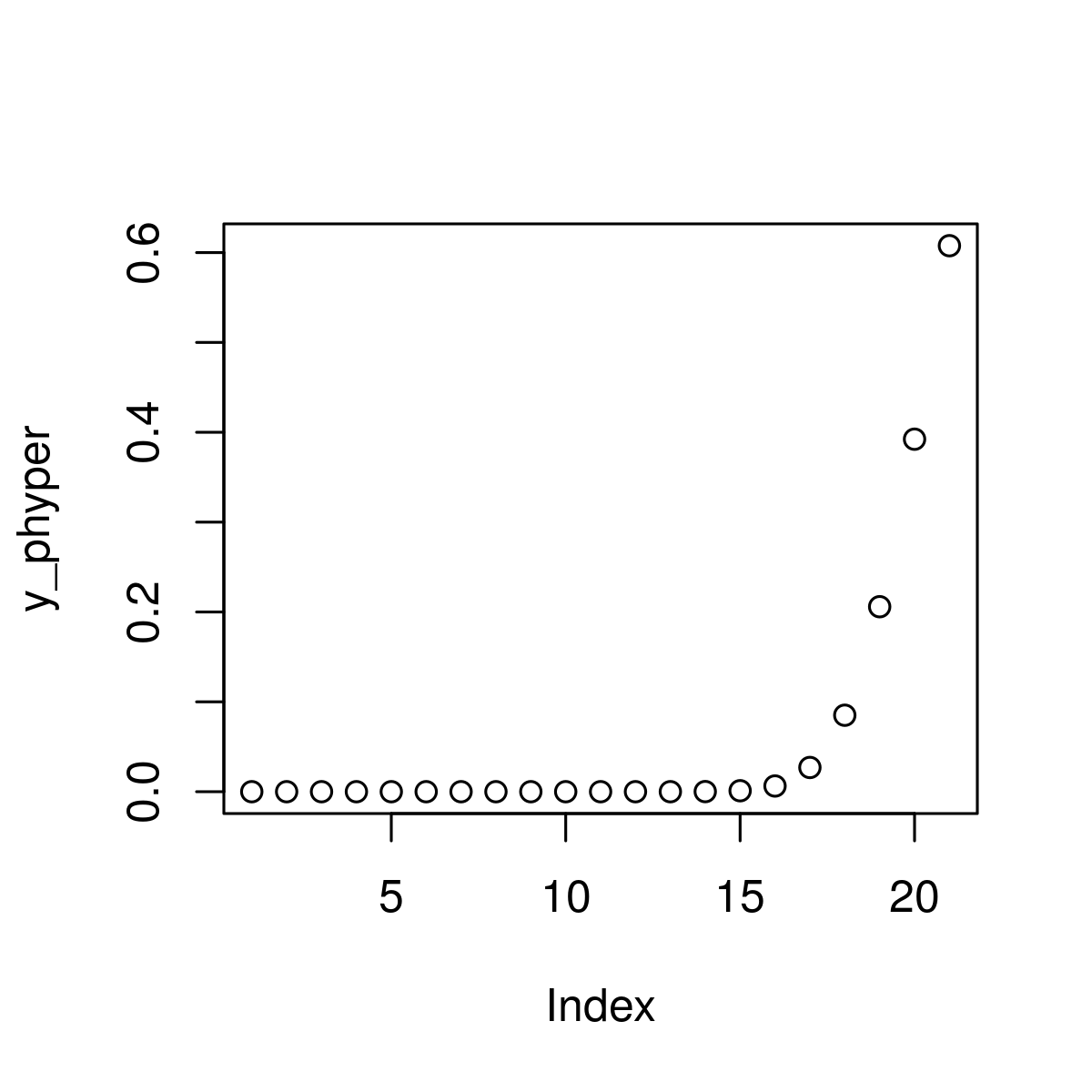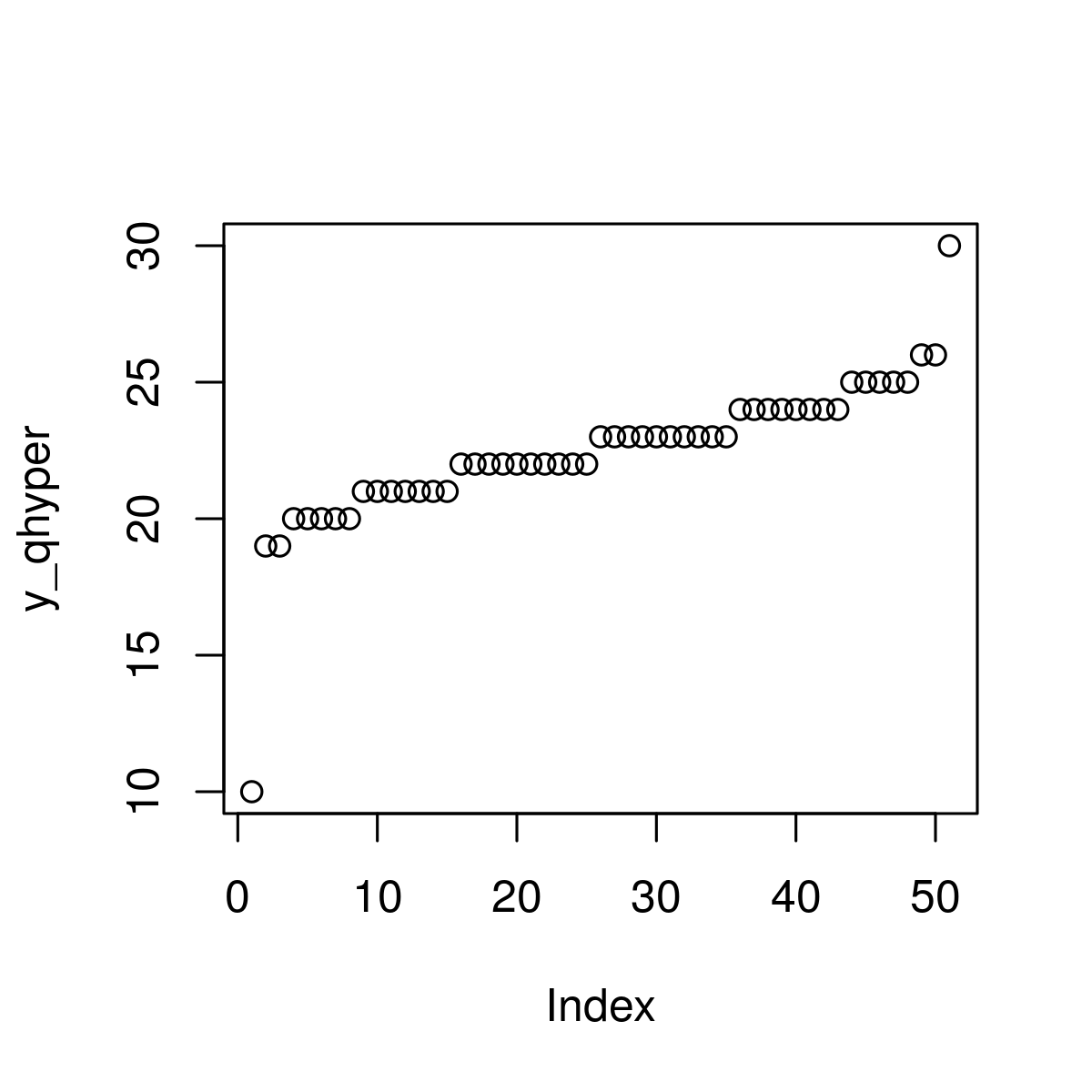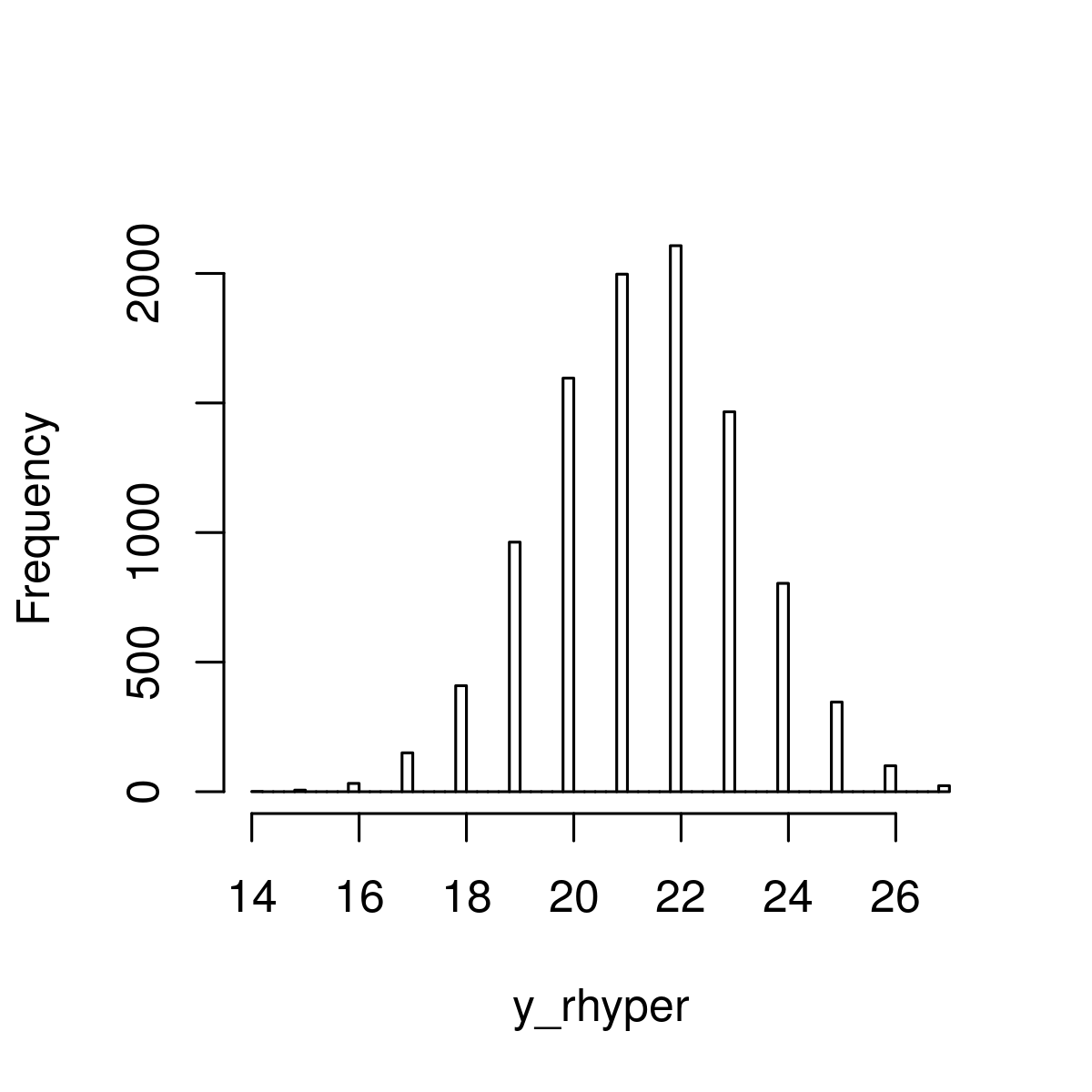Related Articles
Hypergeometric Distribution in R Programming
• Last Updated : 10 Jul, 2020

Hypergeometric Distribution in R Language is defined as a method that is used to calculate probabilities when sampling without replacement is to be done in order to get the density value.

In R, there are 4 built-in functions to generate Hypergeometric Distribution:

• dhyper()
`dhyper(x, m, n, k)`
• phyper()
`phyper(x, m, n, k)`
• qhyper()
`qhyper(x, m, n, k)`
• rhyper()
`rhyper(N, m, n, k)`

where,

x: represents the data set of values
m: size of the population
n: number of samples drawn
k: number of items in the population
N: hypergeometrically distributed values

#### Functions To Generate Hypergeometric Distribution

##### dhyper() Function

It is defined as Hypergeometric Density Distribution used in order to get the density value.

Syntax:

```dhyper(x_dhyper, m, n, k)
```

Example 1:

 `  ` `# Specify x-values for dhyper function``x_dhyper <- ``seq``(0, 22, by = 1.2)`` ` `# Apply dhyper function``y_dhyper <- ``dhyper``(x_dhyper, m = 45, n = 30, k = 20)   ``  ` `# Plot dhyper values``plot``(y_dhyper) `

Output:##### phyper() Function

Hypergeometric Cumulative Distribution Function used estimating the number of faults initially resident in a program at the beginning of the test or debugging process based on the hypergeometric distribution and calculate each value in x using the corresponding values.

Syntax:

`phyper(x, m, n, k)`

Example 1:

 `# Specify x-values for phyper function``x_phyper <- ``seq``(0, 22, by = 1)    ``   ` `# Apply phyper function``y_phyper <- ``phyper``(x_phyper, m = 40, n = 20, k = 31)  `` ` `# Plot phyper values``plot``(y_phyper)  `

Output:##### qhyper() Function

It is basically Hypergeometric Quantile Function used to specify a sequence of probabilities between 0 and 1.

Syntax:

`qhyper(x, m, n, k)`

Example 1:

 `# Specify x-values for qhyper function``x_qhyper <- ``seq``(0, 1, by = 0.02)        `` ` `# Apply qhyper function``y_qhyper <- ``qhyper``(x_qhyper, m = 49, n = 18, k = 30)    `` ` `# Plot qhyper values``plot``(y_qhyper)`

Output:##### rhyper() Function

It generally refers to generating random numbers function by specifying a seed and sample size.

Syntax:

`rhyper(x, m, n, k)`

Example 1:

 `# Set seed for reproducibility``# Specify sample size``set.seed``(400)                                 ``N <- 10000                                      `` ` `# Draw N hypergeometrically distributed values``y_rhyper <- ``rhyper``(N, m = 50, n = 20, k = 30) ``y_rhyper         `` ` `# Plot of randomly drawn hyper density``hist``(y_rhyper,                                          ``     ``breaks = 50,``     ``main = ``""``)`

Output :My Personal Notes arrow_drop_up